Updated by Kim DeBoom on Sep 24, 2015
REPORTKim DeBoom
Owner
27 items   2 followers   0 votes   40 views

# Multiplication Apps

Multiplication apps for the classroom.

27

## Multiplication+: \$Free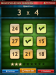The App Gate presents Multiplication Training: an app designed for the iPad to help children of various skill levels practice multiplication from 1x1 to 9x9.
Rules:
1. A question will appear on screen.
2. A correct answer will remove the answer from the screen, and ask the next question in the set.
3. Incorrectly answering a question will reset the current question set.
4. Once the set is complete, a new question set will be drawn randomly based on the current app settings.

26

## Multiplication Menu - Math Games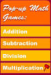Multiplication math games for kids. Learn multiplying in a free, fun way.

25

## Multiplication Menu - Math GamesMultiplication math games for kids. Learn multiplying in a free, fun way.

24

## BBC - KS2 Maths - Multiplication activity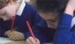An animated KS2 activity for the Maths topic: Multiplication

23

## Around the World | MrNussbaum.com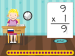Another site to practice facts on.

22

## BBC Bitesize Maths - Factors and multiples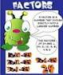Maths number exercise - Factors and multiples activity

21

## ABCya! Division Drag Race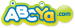Division Drag Race is an educational multi-player racing activity that allows kids to practice their division facts!

20

## Factors Menu - Math Games at Sheppard Software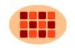Factors, factoring and multiplication games: learn how to find factors with fun math games.

19

## ABCya! Multiplication Grand PrixMultiplication Grand Prix is an educational multi-player racing activity for kids to practice multiplication. Students race against each other to capture the Multiplication Cup!

18

## Rader's NUMBERNUT.COM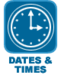Number nut activities are really online quizzes instead of real games. The site is easy to navigate with lots of math skills featured. The home page outlines all the main topics very clearly.

17

## FunBrain.com - Kids Center - Funbrain.com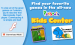Funbrain includes a math arcade among other academic subjects. Games are also arranged by grade level K-8

16

## Math Game Time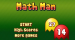Math Game Time has games for preK to seventh grade. Besides being arranged by grade, games are also arranged in a convenient list of skills such as fractions, probability, multiplication, etc. The games are fairly simple in their layout but do provide an engaging format for practicing what might be boring on a worksheet.

15

## Arcademic Skill Builders - Fun Educational Games for Kids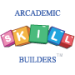FOn this site, the games are neatly organized along a yellow horizontal strip: Addition, Subtraction, Multiplication, Integers, Decimals, Money, Fractions, Time and other non-math topics.

The games are moderately engaging and do a good job of focusing on the math skills being reviewed or taught.

14

## Multiplication Genius Lite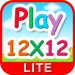Multiplication Genius Lite (Free Lite): This multiplication flash-card based math app is high-interest and skills based learning. Students enjoy the positive reinforcement based format and learn rapidly. I use it in my classroom because it can be custom tailored to focus on specific multiplication facts. This app serves many of the K-6 Common Core State Standards, but here is one primary 3rd grade focus:

Common Core Content Standards Connection:
Operations and Algebraic Thinking 3.OA

Represent and solve problems involving multiplication and division.
1. Interpret products of whole numbers, e.g., interpret 5 × 7 as the total number of objects in 5 groups of 7
objects each, or 7 groups of 5 objects each. For example, describe a context in which a total number
of objects can be expressed as 5 × 7.

13

## Multiplication Number Bubbles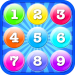This app contains two games--Addition and Multiplication(base on Chinese Multiplication Table).
☞ Addition:Select number bubbles whose sum is equal to the target number.
☞ Multiplication:Select two number bubble whose product is equal to the target number.

12

## Mad Math Lite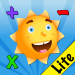Mad Math is perfect for kids to practice their addition, subtraction, multiplication, and Division Facts. Students can even draw right on the flashcard!

11

## Space Math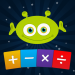Space Math is fun way for kids to practice their addition, subtraction, multiplication, and division facts.

10

## Multiplication+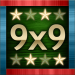Multiplication Training: an app designed for the iPad to help children of various skill levels practice multiplication from 1x1 to 9x9. Multiplication sets are completely configurable: any subset of 1x-9x can be turned on or off. Question sets can be configured to appear in sequential order (2x1, 2x2, 2x3…) for novice students or can be configured to be random (2x9, 2x4, 2x3…) for advanced students. iPad only app.

9

## Motion Math: Wings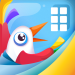Master Multiplication with Flying Colors! Practice mental multiplying and estimation with Motion Math: Wings! This fun math game helps children ages 4 and up develop a conceptual understanding using six different visual forms. It's simple to play - you just tilt your bird to the bigger number. Universal app.

8

## Math Vs Zombies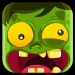Save Yourself from a Zombie Attack using Math as Your Weapon! Math practice for addition, subtraction, multiplication, and division. Universal app.

7

## Sushi MonsterStrengthen reasoning strategies for whole number addition and multiplication by helping monsters make a target sum or product. Earn points with each correct answer… but watch out for distractions! To be successful, plan ahead and strategically select numbers from the sushi counter. Universal app.

6

## Thinking Blocks Multiplication and Division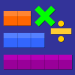Thinking Blocks Multiplication teaches children how to model and solve word problems involving multiplication and division. In this interactive tutorial, children are introduced to 6 problem solving models. The models help children organize information and visualize number relationships.

5

## Multiplication Genius x19 Free By Blue Onion Soft, Inc.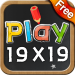MATH
Free
4+
Encourage your kids to master 9, 12 and 19 Times Table! The multiplication is the most The challenge is 'MULTIPLICATION x19' Tip: Test your friends how smart they are and see if they can beat you. ;)

4

## Multiplication Plus By asunaroseven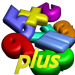MATH
free
4+
This is software for exercising multiplication.With the random question mode, you chosse the number of question. And you push start button. So you are given questions.When you answer all the questions,it is displayed correct answer and your time.Accuracy rate is displayed in the random question mode.

3

## Multiplication Genius LiteMultiplication Genius Lite (Free Lite): This multiplication flash-card based math app is high-interest and skills based learning. Students enjoy the positive reinforcement based format and learn rapidly. I use it in my classroom because it can be custom tailored to focus on specific multiplication facts. This app serves many of the K-6 Common Core State Standards, but here is one primary 3rd grade focus:

Common Core Content Standards Connection:
Operations and Algebraic Thinking 3.OA

Represent and solve problems involving multiplication and division.
1. Interpret products of whole numbers, e.g., interpret 5 × 7 as the total number of objects in 5 groups of 7
objects each, or 7 groups of 5 objects each. For example, describe a context in which a total number
of objects can be expressed as 5 × 7.